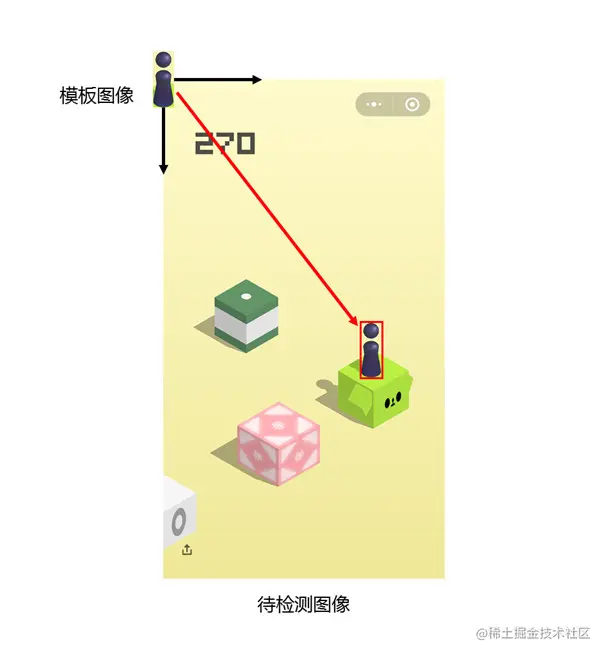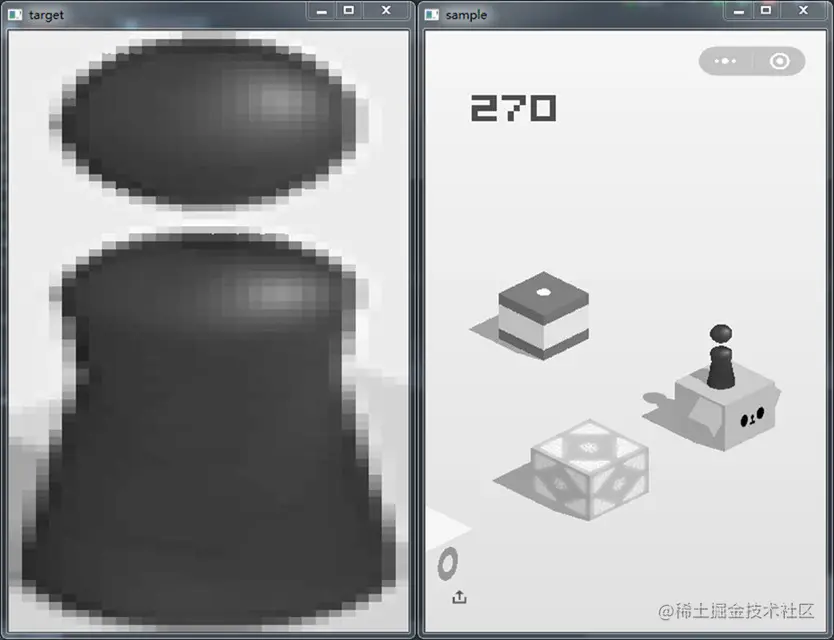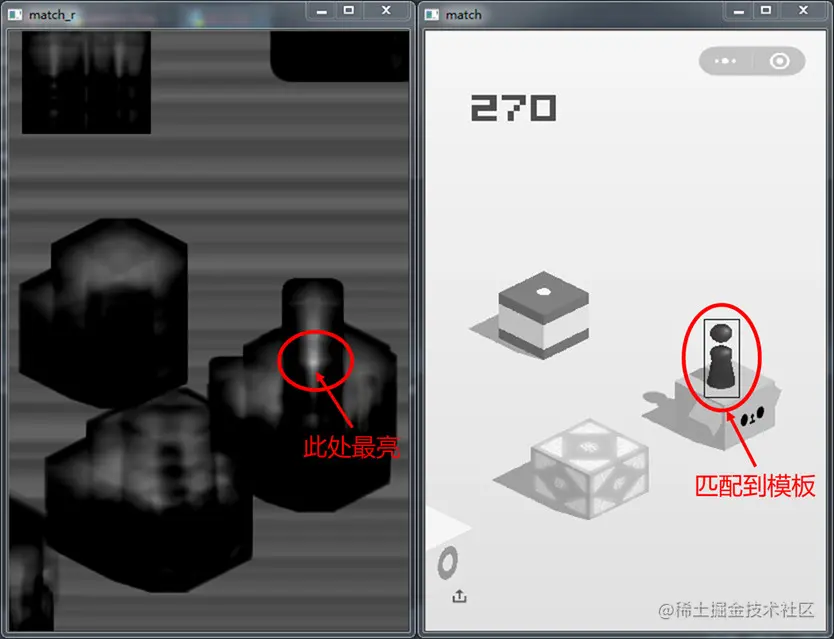# Python image processing, CV2 module, OpenCV to achieve template matching

2022-02-02 03:57:32

Time is not negative , Create constantly , This article is participating in 2021 Year end summary essay contest

# Preface

utilize Python Realization OpenCV Achieve edge detection . I don't say much nonsense .

Let's start happily ~

# development tool

Python edition ： 3.6.4

Related modules ：

cv2 modular ;

As well as some Python Built in modules .

# Environment building

install Python And add to environment variable ,pip Install the relevant modules required .

# Template matching

Template matching , It is to find small areas matching the given sub image in the whole image area

We need a template image here ( Given the subimage ) And an image to be detected ( Original image )

On the image to be detected , From left to right , The matching degree between template image and overlapping sub image is calculated from top to bottom , The greater the matching degree is , The more likely they are to be the sameThis is mainly about OpenCV Of cv2.matchTemplate() and cv2.minMaxLoc() function

The first function is to find a match between the template and the input image , Get the matching result image

The second function is to find the maximum and minimum values in a given matrix ( Including their location )

There are six kinds of template matching algorithms

``````#  The first category , Using the square difference to match , The best match is 0. Match the worse , The larger the match value

#  Square difference matching
method=CV_TM_SQDIFF

#  Standard square difference matching
method=CV_TM_SQDIFF_NORMED

#  The second category , The multiplication between template and image is used , So a larger number means a better match ,0 Identify the worst matching effect

#  Correlation matching
method=CV_TM_CCORR

#  Standard correlation matching
method=CV_TM_CCORR_NORMED

#  The third category , The relative value of the template to its mean value is matched with the correlation value of the image to its mean value ,1 A perfect match ,-1 A bad match ,0 There is no correlation ( Random sequence )

#  Correlation coefficient matching
method=CV_TM_CCOEFF

#  Standard correlation coefficient matching
method=CV_TM_CCOEFF_NORMED
Copy code ``````

Standardization means unifying values to 0~1

# Retrieve image

Look at the two images , It's all grayscale

``````import cv2

#  Read the image to be detected
img = cv2.imread('game.png', 0)
#  Read template image
temple = cv2.imread('temple.png', 0)

#  Display the image to be detected after grayscale processing
cv2.namedWindow('sample', 0)
cv2.resizeWindow('sample', 400, 600)
cv2.imshow('sample', img)

#  Display the template image after grayscale processing
cv2.namedWindow('target', 0)
cv2.resizeWindow('target', 400, 600)
cv2.imshow('target', temple)
Copy code ``````

Result displayThe first is a template image , The second one is the image to be detected

Use OpenCV Two functions of , To achieve template matching

``````#  Get the height and width of the template image
th, tw = temple.shape[:2]
print(th, tw)

#  Using standard correlation coefficients to match ,1 A perfect match ,-1 A bad match ,0 There is no correlation
result = cv2.matchTemplate(img, temple, cv2.TM_CCOEFF_NORMED)

# result To match the result matrix
# print(result)

# TM_CCOEFF_NORMED Method after processing the result image
cv2.namedWindow('match_r', 0)
cv2.resizeWindow('match_r', 400, 600)
#  Display window
cv2.imshow('match_r', result)

#  Using functions minMaxLoc, Determine the maximum and minimum values of the matching result matrix (val), And where they are (loc)
min_val, max_val, min_loc, max_loc = cv2.minMaxLoc(result)

#  Select the position of the maximum value here , For the upper left corner of the image
tl = max_loc
#  Get the lower right corner of the image
br = (tl+tw, tl+th)
#  Draw rectangle
cv2.rectangle(img, tl, br, (0, 0, 255), 2)

#  Set the display window
cv2.namedWindow('match', 0)
cv2.resizeWindow('match', 400, 600)
#  Display window
cv2.imshow('match', img)

#  end
cv2.waitKey(0)
cv2.destroyAllWindows()
Copy code ``````

Results outputThe whitest position in the first picture , That means the highest match

Second figure , The rectangle represents the result of the match

Through the location parameters of the rectangular box , Combine the size of the template image , You can get the center point of checkers ( Bottom )